# NHÀ khoa học nguyễn văN ĐẠo với lý thuyết dao đỘng và chuyểN ĐỘng hỗN ĐỘN

tải về 3.08 Mb.
 trang 11/11 Chuyển đổi dữ liệu 15.11.2017 Kích 3.08 Mb.
 A. It is the change in length in this direction that the largest Lyapunov exponent measures. After a given time interval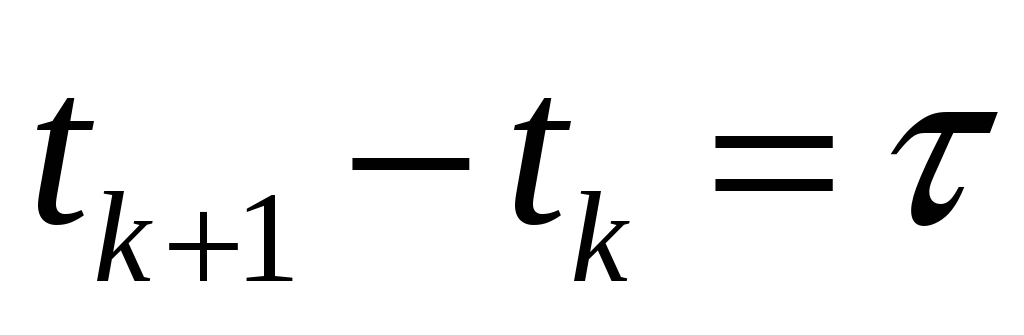, take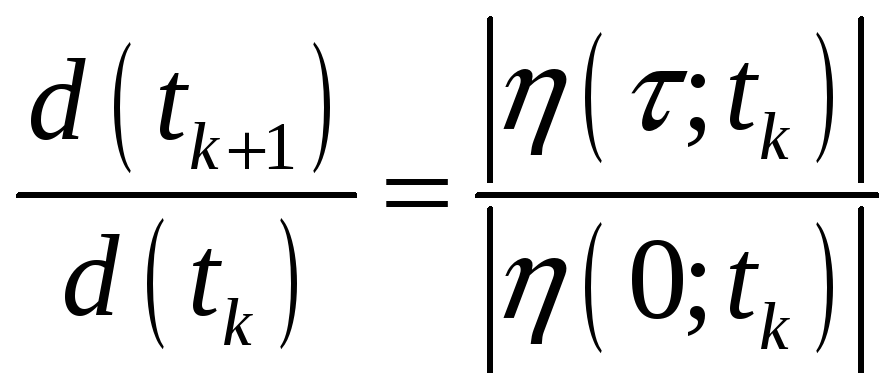The time evolution of the Lyapunov exponent is presented in Fig.14. The largest Lyapunov exponent value is a positive number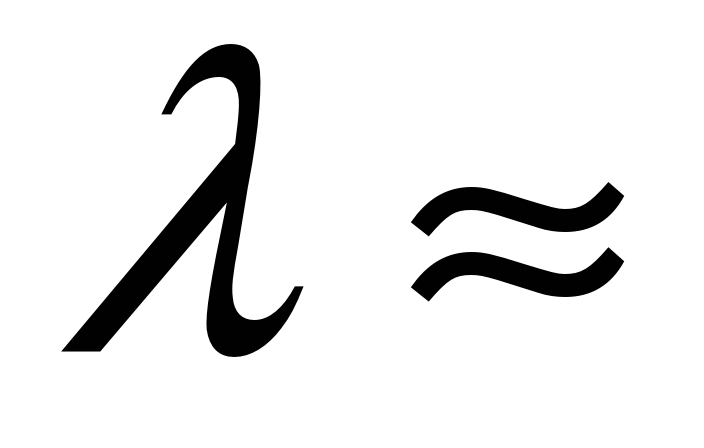0.0698>0, which shows the chaotic character of the motion of the system (10). This means that two trajectories starting closely one to another in the phase space will move exponentially away from each other for small times on the average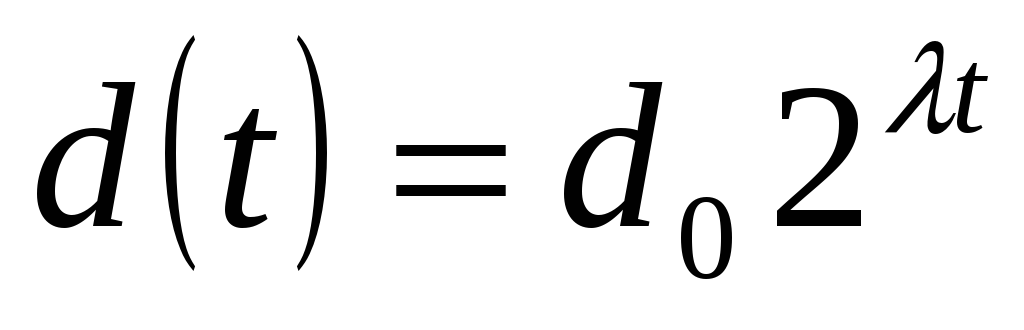, where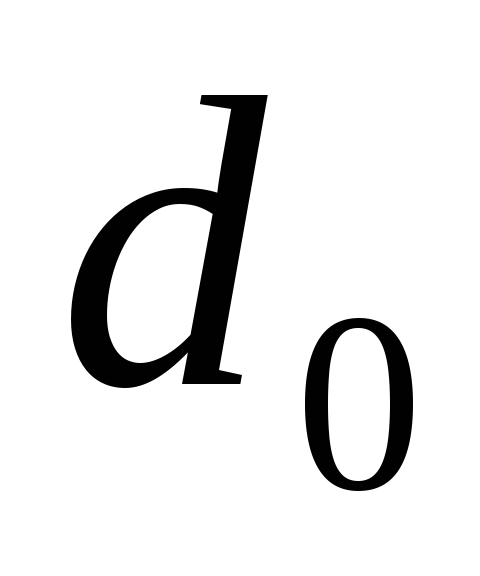is the initial distance between two adjacent starting points at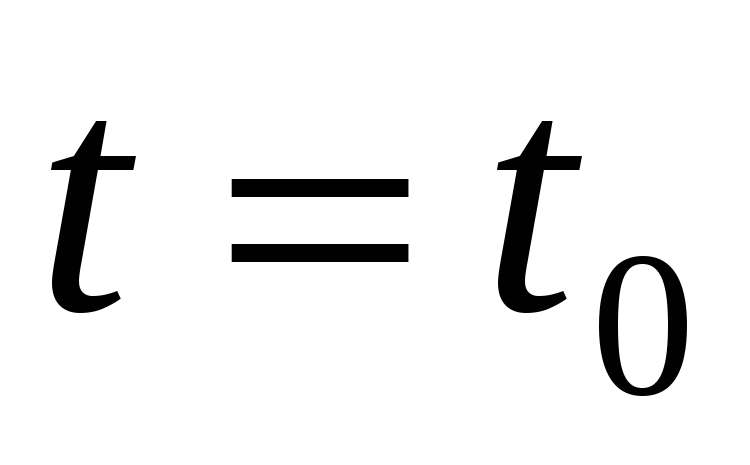and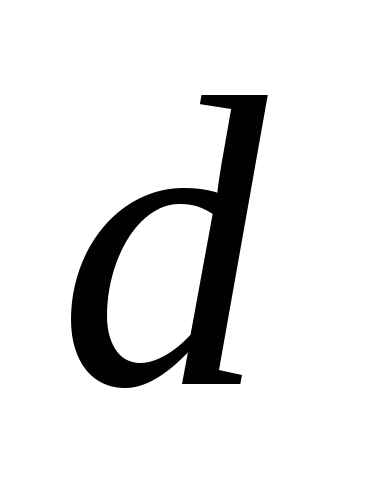is the distance between two these points at the moment. In Fig. 15, we show how the distancebetween evolutions initiated from two points separated by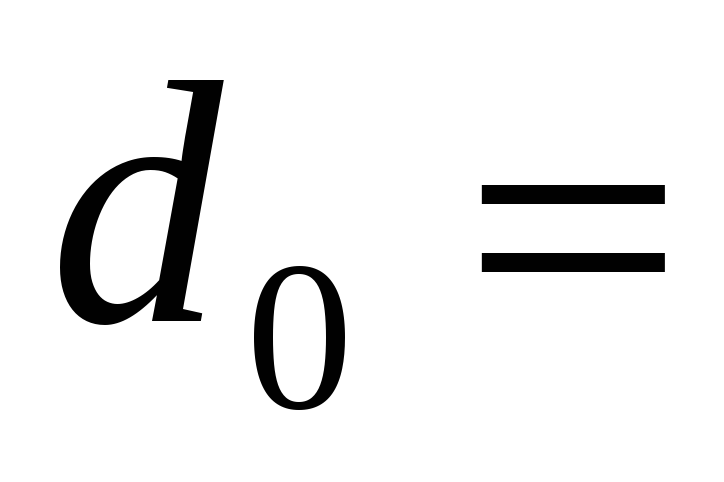10-7 varies with time. Both of the initial points are located close to the attractor. The separation clearly grows exponentially in the range 10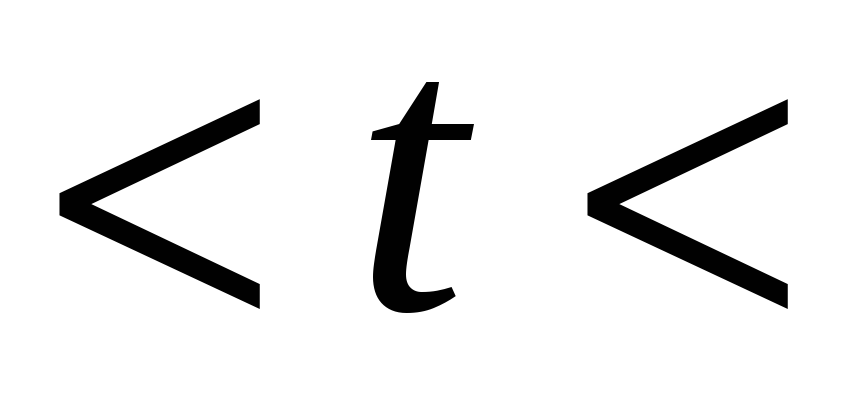125. The separation saturates at the size of the attractor for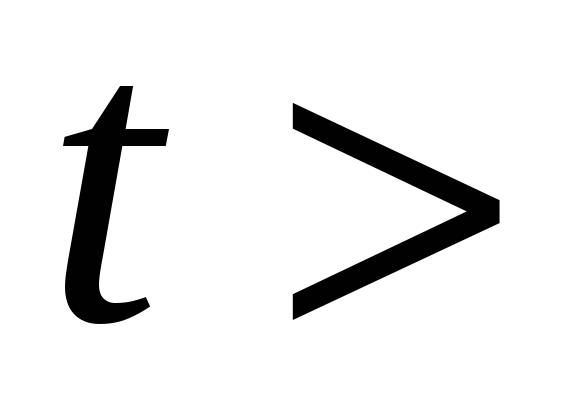125.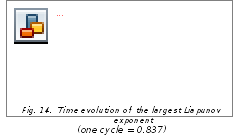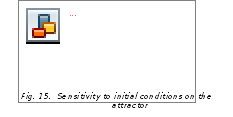The bifurcation diagrams and Liapunov exponent have been used for detecting chaotic regimes in the system described by equation (1). Our discussion was focused upon variation of the excitation frequencyand the forcing amplitude e . For a concrete case, the parameter regions in which either periodic or chaotic motions exist were shown. Chaotic attractors (Fig. 5, 10) illustrate the complexity of the motion of the system under consideration. The bifurcation diagram shows so clearly the motions of system (1) with respect to the observed parameters. In two preceding cases, the first case, whenis control parameter, it changes suddenly from periodic motion to chaotic motion, corresponding to Hopf bifurcation. In the second case, it is the double – period process and leads to chaotic motion. Lyapunov exponent value and some other criterions have used to determine chaotic motion of a solution. * * * Công trình khoa học này của Anh là báo cáo mời, sẽ báo cáo vào ngày thứ hai, 11/12/2006 tại Hội nghị Quốc tế “Giải tích phi tuyến và Cơ học ngày nay” từ ngày 11 đến 14/12/2006 tại thành phố Hồ Chí Minh. Nhưng Anh đã ra đi mãi mãi vào chính buổi sáng hôm đó. Sự nghiệp khoa học thực sự đã đi theo Anh đến hơi thở cuối cùng. Khi chuẩn bị báo cáo này chẳng ai ngờ rằng đây là báo cáo cuối cùng của Anh. Hà Nội, 29/7/2007

Cơ sở dữ liệu được bảo vệ bởi bản quyền ©hocday.com 2019
được sử dụng cho việc quản lý

Quê hương